# Kernels vs. Filters: Demystified

#### Understanding the difference once and forever.Photo by bruce mars on Unsplash

For most of us, who were once newbies in Deep Learning, trying tf.keras.layers.Conv2D for MNIST classification was fun. Convolutions are the building blocks of most algorithms in computer vision, except for some newer variants like Vision Transformers, Mixers, etc. which claim to solve image-related problems without the use of convolutions. At the core of DL, lies Gradient Descent ( and its variants ), which helps us optimize the parameters of a NN, which in turn reduces the loss we incur while training the model.

Convolutions or Convolutional layers also possess their own parameters commonly known as filters. No, not filters but they are kernels, right? Still confused, well, that’s the aim of the story!

###Refresher on Convolutions

As described in most deep learning literature, a *2D convolutional layer takes in a tensor of shape ( h , w , in_dims ) and produces a feature map, which has a shape ( h' , w' , out_dims ) . In case there’s no *padding, h' and w' are smaller than h and w respectively. You might have come across this popular animation depicting a typical convolution operation on a single-channeled ( in_dims=1) square ( h=w) image.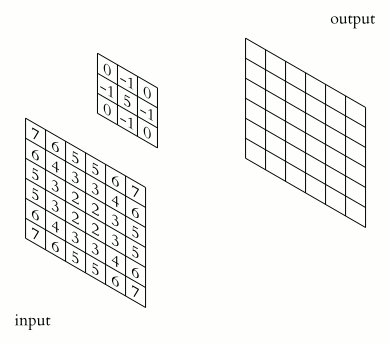Convolution on a single channeled input. Source: Wikimedia Commons

In the case of a 2D convolution, the matrix containing some numbers, called the kernel, moves across the image. We typically set the kernel_size and strides here. The kernel_size determines the size of the kernel whereas the strides is the number of pixels ( values ) the kernel moves in one particular direction, to perform the multiplication. The values from the input tensor are then multiplied with the corresponding values in the kernel in an elementwise fashion and finally summed up to produce a scalar. The kernel then moves ahead ( according to strides ) and performs a similar problem.

These scalars are then arranged in a 2D grid in a way just as they were obtained. We would then apply some activation function over it such ReLU.

*2D convolutional layer: We also have 1D and 3D convolutions where the kernel moves in 1 or 3 dimensions respectively. See this intuitive story. As 2D convolutions are widely used, and they can be visualized easily, we’ll consider studying the case of 2D convolutions only.

*padding: Since, the convolution operation reduces the dimensions of the input tensor, we would like to restore the original dimensions by bordering it with zeros. Padding performed with zeros is called zero padding in ML land.

###The Kernel

The kernel is that matrix which is swiped, or more precisely convolved across a single channel of the input tensor.

Using the same diagram, as we used earlier,Convolution on a single channeled input. Source: Wikimedia Commons

In the above representation, one can clearly observe the 3 * 3 kernel being convolved across a single-channeled input tensor. In most implementations, the kernel is a square matrix which no. of columns ( and rows ) equal to the kernel_size , but can be rectangular, as well. That’s exactly the TensorFlow docs say for their tf.keras.layers.Conv2D layer,

kernel: An integer or tuple/list of 2 integers, specifying the height and width of the 2D convolution window. Can be a single integer to specify the same value for all spatial dimensions.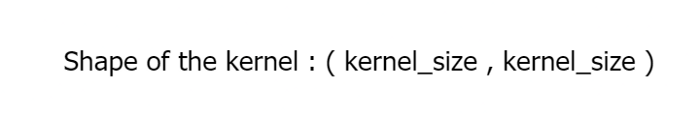The shape of the kernel ( where height = width ). Source: Image By Author

After performing the convolution operation, we’re left with a single channeled feature map, as described earlier. But, there’s a problem. Most input tensors won’t have a single channel. Even if we are performing the convolution operation on an RGB image, we need to process 3 channels ( i.e. the R, G, and B channels ). But, we need not worry, as we can simply use 3 kernels for the 3 channels, right?

That’s for 3 channels, but what if we have multiple input channels, say 256 channeled tensors? Using the same analogy, we’ll have 256 kernels convolving each of the 256 channels and thus producing 256 feature maps ( which, as assumed earlier, have a smaller size ). All those 256 feature maps are then added together, like,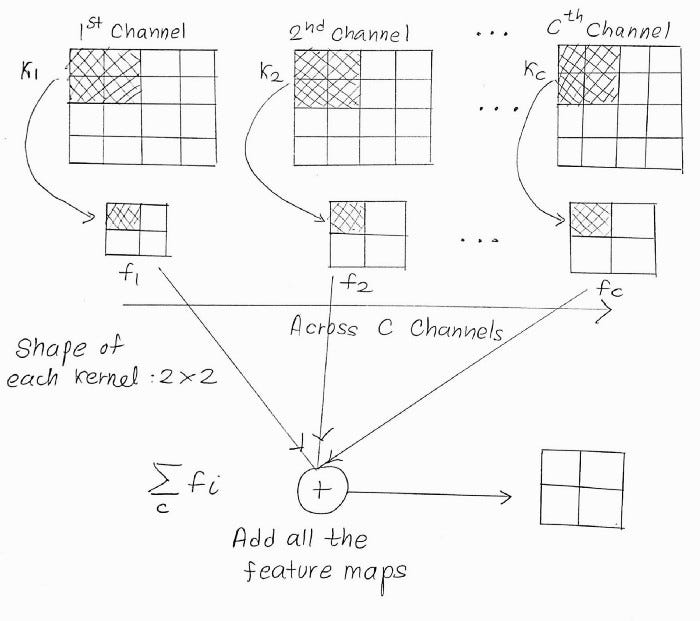Convolving kernels across multiple channels. Source: Image By Author.

If C_in is the number of input channels, then,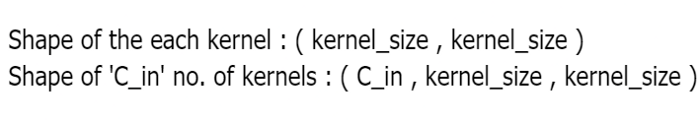Source: Image By Author

This is all about the kernel! We shall now head towards the filter.

###The Filter

The collection of all kernels which are convolved on the channels of the input tensor.

A filter is the collection of all C_in no. of kernels used in the convolution of the channels of the input tensor. For instance, in an RGB image, we used 3 different kernels for the 3 channels, R, G, and B. These 3 kernels are collectively known as a filter. Hence, the shape of a single filter is,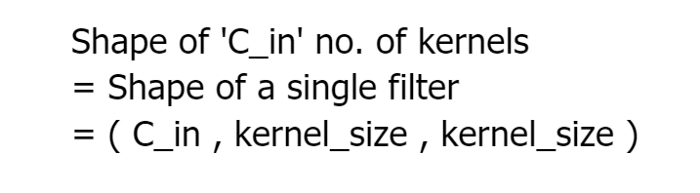Image by Author

Let’s get back to the TensorFlow docs for the tf.keras.layers.Conv2D layer. They include the following example for this layer,

input_shape = (4, 28, 28, 3)
conv = tf.keras.layers.Conv2D( 2, 3, activation='relu', input_shape=input_shape[1:])(x)

For the first argument while instantiating Conv2D , the description given is,

filters: Integer, the dimensionality of the output space (i.e. the number of output filters in the convolution).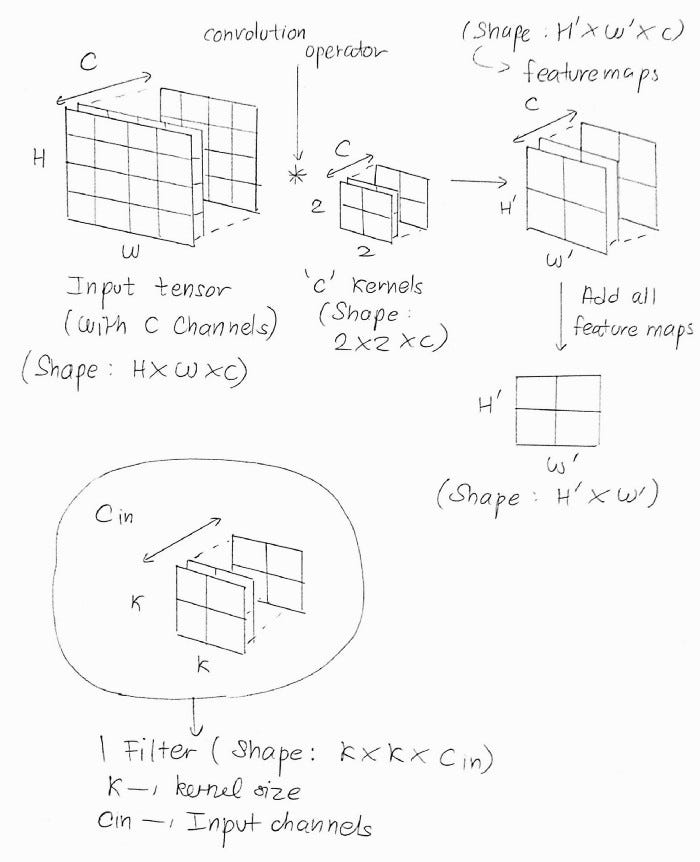Source: Image By Author

Each filter would produce a feature map of the shape H' * w'. Likewise, filters a number of filters would produce filters filters. Hence the output shape of the Conv2D layer is ( H' , W' , filters ) . That’s the same output shape mentioned in the TensorFlow docs of tf.keras.layers.Conv2D ,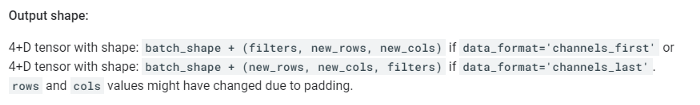Source: Screenshot from TensorFlow Documentation.

That brings us to the end of this story. Hope you’ve understood the difference between the two terms.

### More from the Author

https://medium.com/media/4f4b80390d40a588b8598760a90795ba/hrefhttps://medium.com/media/e162bb093d5fba8d663293102a4d5c92/href

### The End

Hope you enjoyed this short story! Feel free to express your thoughts at equipintelligence@gmail.com or in the comments below. Have a nice day ahead!Kernels vs. Filters: Demystified was originally published in Towards AI on Medium, where people are continuing the conversation by highlighting and responding to this story.

Published via Towards AI